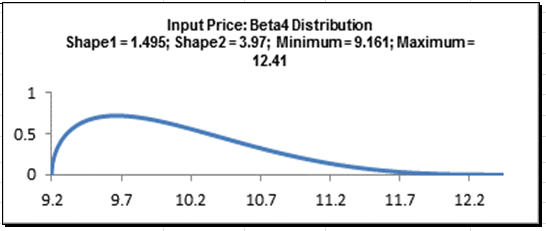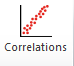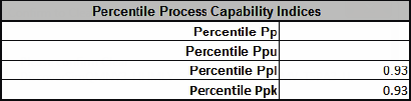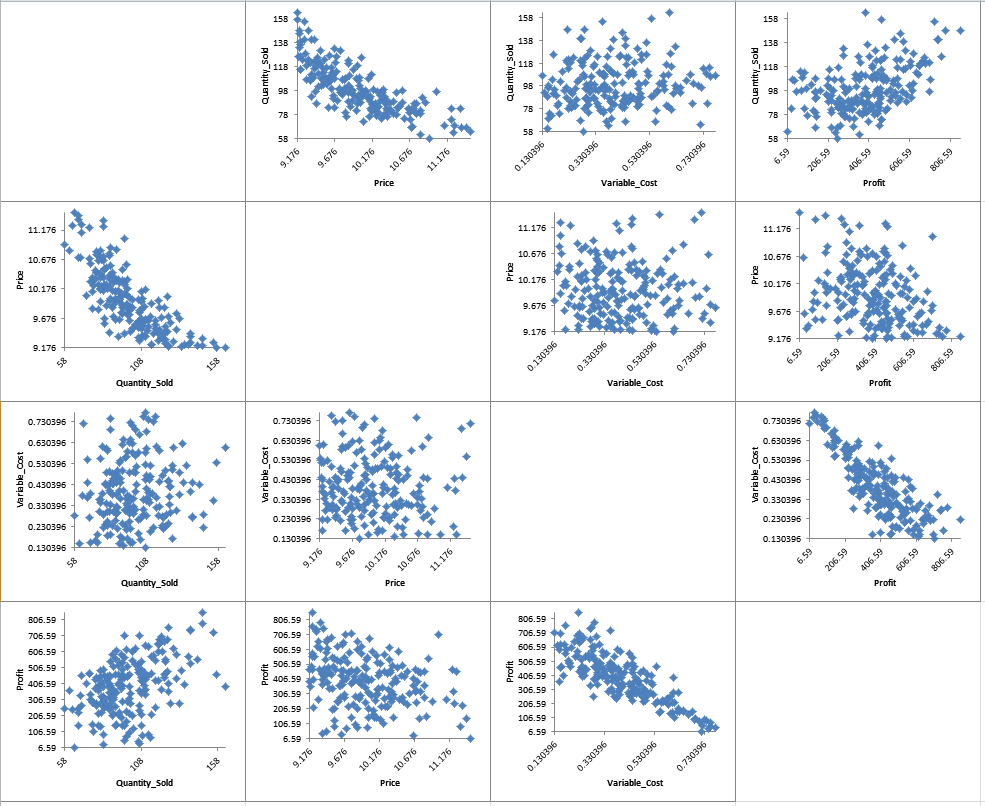Include Top

# DiscoverSim™ Case Studies

Back to Table of Contents

## Case Study 1 – Basic Profit Simulation

1. Open the workbook Profit Simulation. DiscoverSim Input Distributions will simulate the variability in Quantity Sold, Price and Variable Cost. We will use distribution fitting with historical data to determine which distributions to use and what parameter values to enter. Input Distributions will then be specified in cells C11, C12 and C13. The output, Profit, will be specified at cell C21 using the formula given above.

2.3. Select the Historical_Data sheet. This gives historical data for Quantity Sold, Price and Variable Cost.

4.Tip: At this point use the SigmaXL tool (bundled with DiscoverSim) to obtain descriptive statistics to test for normality (SigmaXL > Statistical Tools > Descriptive Statistics). Quantity Sold has an Anderson Darling Normality test p-value = 0.83, so may be considered as a Normal Distribution, but since it is a count we will apply DiscoverSim’s distribution fitting using discrete distributions. Price and Variable Cost are non-normal with AD p-values less than .05, so we will use DiscoverSim’s distribution fitting for continuous data. SigmaXL’s correlation matrix should also be used to evaluate correlations (SigmaXL > Statistical Tools > Correlation Matrix). The Spearman Rank correlation for Quantity Sold versus Price is -0.8 (Note: DiscoverSim uses the more robust Spearman Rank correlation rather than Pearson’s correlation). SigmaXL’s graphical tools such as Histograms and Scatterplots should also be used to view the historical data.

5. Select DiscoverSim > Distribution Fitting:

6.7. The data range has been preselected and appears in the dialog. Note: if a different range is required, click onto change it or select Use Entire Data Table to automatically select the data.

8.9. Click Next. Select Quantity Sold as the Numeric Data Variable (Y). Since this is count data, i.e. a discrete variable, select Discrete. For Distribution Options use the default All Discrete Distributions as shown:

10.11. Click OK. The resulting discrete distribution report is shown below:

12.Since these are discrete distributions, Chi-Square is the statistic used to determine goodness-of-fit. The distributions are sorted by Chi-Square in ascending order. The best fit distribution is Negative Binomial with Chi-Square = 35.4 and p-value = “> .5”. The parameters are Number of Required Events = 33 and Event Probability = 0.2534. (See Appendix for further details on distributions and distribution fitting).

Tip: If the best fit discrete distribution has a p-value less than < .05 indicating a poor goodness-of-fit, none of the discrete distributions are adequate for use in Monte-Carlo simulation. In this case you should redo the distribution fit using the Continuous option (Note that you can use the continuous option for discrete data, but you cannot use discrete distributions for continuous data). After creating a DiscoverSim input distribution with the best fit (or normal if applicable), use Excel’s ROUND(number, 0) function to obtain integer values from the continuous distribution.

13. Now we will apply distribution fitting to Price and Variable Cost. Select the Historical_Data sheet and repeat the above steps. Both variables are continuous so use the default Select Distribution Type Continuous. The resulting distribution reports are shown below.

14.15. DiscoverSim uses the Anderson Darling statistic to determine goodness-of-fit for continuous distributions. The distributions are sorted by the AD statistic in ascending order. The best fit distribution for Price is Beta (4 Parameters) with AD Stat = .34 and AD p-value = 0.49. The best fit distribution for Variable Cost (%) is Johnson SB with AD Stat = .27 and AD p-value = “> .5”.
16. Select Profit_Model sheet. Click on cell C11 to specify the Input Distribution for Quantity Sold. Select DiscoverSim > Input Distribution:

17.18. Click Select Stored Distribution Fit. We will use the default variable Quantity Sold for Select Distribution Fit Variable and Negative Binomial for Select Distribution. The parameter values for Number of Required Events and Event Probability are automatically populated from the distribution fit results for Quantity Sold.
19. Enter “Quantity_Sold” as the Input Name.
20. Click Update Chart. The completed Create/Edit Input Distribution dialog is shown below:

21.22. Click OK. Hover the cursor on cell C11 in the Profit_Model sheet to view the DiscoverSim graphical comment showing the distribution and parameter values:
23. Click on cell C12 to specify the Input Distribution for Price. Select DiscoverSim > Input Distribution.
24. Click Select Stored Distribution Fit. Select the variable Price for Select Distribution Fit Variable and the default Beta (4 Parameters) for Select Distribution. The parameter values for this distribution are automatically populated from the distribution fit results for Price.
25. Enter “Price” as the Input Name.
26. Click Update Chart. The completed Create/Edit Input Distribution dialog is shown below:

27.28. Click OK. Hover the cursor on cell C12 in the Profit_Model sheet to view the DiscoverSim graphical comment showing the distribution and parameter values:

29.30. Click on cell C13 to specify the Input Distribution for Variable Cost. Select DiscoverSim > Input Distribution.
31. Click Select Stored Distribution Fit. Select Variable Cost for Select Distribution Fit Variable and the default Johnson SB for Select Distribution. The parameter values for this distribution are automatically populated from the distribution fit results for Variable Cost.
32. Enter “Variable_Cost” as the Input Name.
33. Click Update Chart. The completed Create/Edit Input Distribution dialog is shown below:

34.35. Click OK. Hover the cursor on cell C13 in the Profit_Model sheet to view the DiscoverSim graphical comment showing the distribution and parameter values:

36.37. Now we will specify the correlation between the inputs Quantity Sold and Price. Select DiscoverSim > Correlations:

38.39. As discussed above, the Spearman Rank correlation between Quantity and Price is -.8. This negative correlation is expected: as order quantity increases, unit price decreases. Enter the value -.8 in the column Price, row Quantity and press Enter. The “mirror” value in the column Quantity, row Price will automatically be populated as shown.

40.Click OK.

41. Now we will specify the Profit model output. Click on cell C21. Select DiscoverSim > Output Response:
42.43. Enter the output Name as “Profit”. Enter the Lower Specification Limit (LSL) as 0. The Weight and Output Goal settings are used only for multiple response optimization, so do not need to be modified in this example.

44.Click OK.

45. Hover the cursor on cell C21 to view the DiscoverSim Output information.

46.47. Select DiscoverSim > Run Simulation:

48.49. Click Report Options/Sensitivity Analysis. Check Percentile Report, Scatter Plot/Correlation Matrix, Sensitivity Regression Analysis, Sensitivity Charts - Correlation Coefficients and Regression Coefficients. Select Seed Value and enter “12” as shown, in order to replicate the simulation results given below.

50.Click Run

51. The DiscoverSim Output Report shows a histogram, normal probability plot, descriptive statistics, process capability indices, a percentile report and a percentile process capability report:

52.From the histogram and detailed report we see that typically we should expect a positive daily profit, but the variation is large. The likelihood of profit loss is approximately 0.56% (see Actual Performance (Empirical): %Total (out of spec)). Note that the “expected” loss of 1.49% assumes a normal distribution, so that is not applicable here because the output distribution is not normal (Anderson-Darling p-value is much less than .05).

Note: If Seed is set to Clock, there will be slight differences in the reported values with every simulation run due to a different starting seed value derived from the system clock

Tip: Percentile Process Capability Indices for non-normal data can be calculated from the Percentile Report as follows:

Percentile Pp = (USL – LSL)/( 99.865 percentile – 0.135 percentile)

Percentile Ppu = (USL – 50th percentile)/(99.865 percentile – 50th percentile)

Percentile Ppl = (50th percentile – LSL)/(50th percentile – 0.135 percentile)

Percentile Ppk = min(Ppu, Ppl)

Since we only have a lower specification limit (LSL = 0), Percentile Ppl is calculated as:

Percentile Ppl = (389.33 - 0) / (389.33 - (-30.81)) = 0.93

53. Click on the Scatter Plot Matrix sheet to view the Input-Output relationships graphically:

54.Here we see the negative correlation that was specified between the inputs “Price” and “Quantity Sold”, as well as the strong negative correlation between “Variable Cost” and “Profit”.

55. Click on the Correlation Matrix sheet to view the Input-Output correlations numerically:

56.DiscoverSim uses the robust Spearman Rank correlation but the Pearson is also reported here for reference purposes. The correlations highlighted in red are statistically significant (p-value < .05).

57. In order to increase profit (and reduce the variation), we need to understand what is driving profit, i.e., the key “X” factor. To do this we will look at the sensitivity charts. Click on the Sensitivity Chart sheet:

58.Here we see that “Variable Cost” is the dominant input factor affecting “Profit” with a negative correlation (lower variable cost means higher profit). The next step would then be to find ways to minimize the variable cost (and reduce the variation of variable cost). “Quantity Sold” is the second important input factor. It is interesting to note that “Price” is the least important factor in this Profit simulation model.

59. To view R-Squared percent contribution to variation, click on the Sensitivity Regression sheet:

60.61. When strong correlations are present in the inputs, Sensitivity Correlation Analysis and Sensitivity Regression Analysis may be misleading, so it is recommended that the simulation be rerun with Independence (Ignore Correlations) checked to validate the sensitivity results:

62.In this case the input factor prioritization remains the same, but “Price” shows a small positive correlation rather than negative.

63. The revised R-Square Percent Contribution report is shown below:

64.Back to Table of Contents

# Web Demos

Our CTO and Co-Founder, John Noguera, regularly hosts free Web Demos featuring SigmaXL and DiscoverSim
Click here to view some now!

# Contact Us

Ph: 1.888.SigmaXL (744.6295)

Support: Support@SigmaXL.com

Sales: Sales@SigmaXL.com

Information: Information@SigmaXL.com